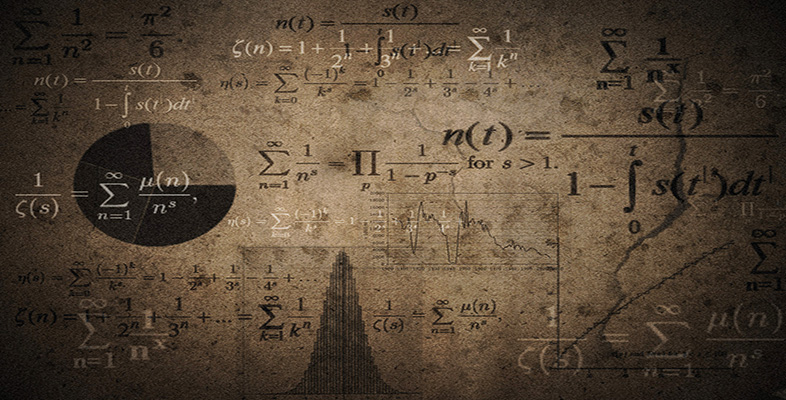Language, notation and formulas

Start this free course now. Just create an account and sign in. Enrol and complete the course for a free statement of participation or digital badge if available.

Free course

# 2.7 Powers and roots

There are several symbols for powers and roots: for instance, 24 means ‘2 to the power 4’. An alternative to 24 is 24, where the symbolmeans ‘to the power’. This symbol is often used by computers and calculators.

A related symbol is the square root symbol, as in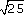meaning the positive square root of 25 (that is, 5). Symbols for other roots, are exemplified by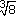meaning the cube root of 8 (that is, 2).

Raising to the power 1 gives the symbol for a reciprocal: thus, 6−1 means the reciprocal of 6, or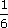. The symbols used by calculators for scientific notation often involve the letter E: for example, 3.1E2 means 3.1 × 102, or 310.

## Example 5

What do the following expressions mean?

• (a) 52

• (b)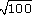• (c) (5 + 8)2

• (d) 2−1

• (e)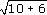• (a) Square 5. (This gives 25.)

• (b) Positive square root of 100 (This gives 10.)

• (c) Add 5 and 8, and then square the result. (This gives 169.)

• (d) The reciprocal of 2. (This givesor 0.5.)

• (e) The square root of the sum of 10 and 6. (That is, 4.)

MU120_4M6